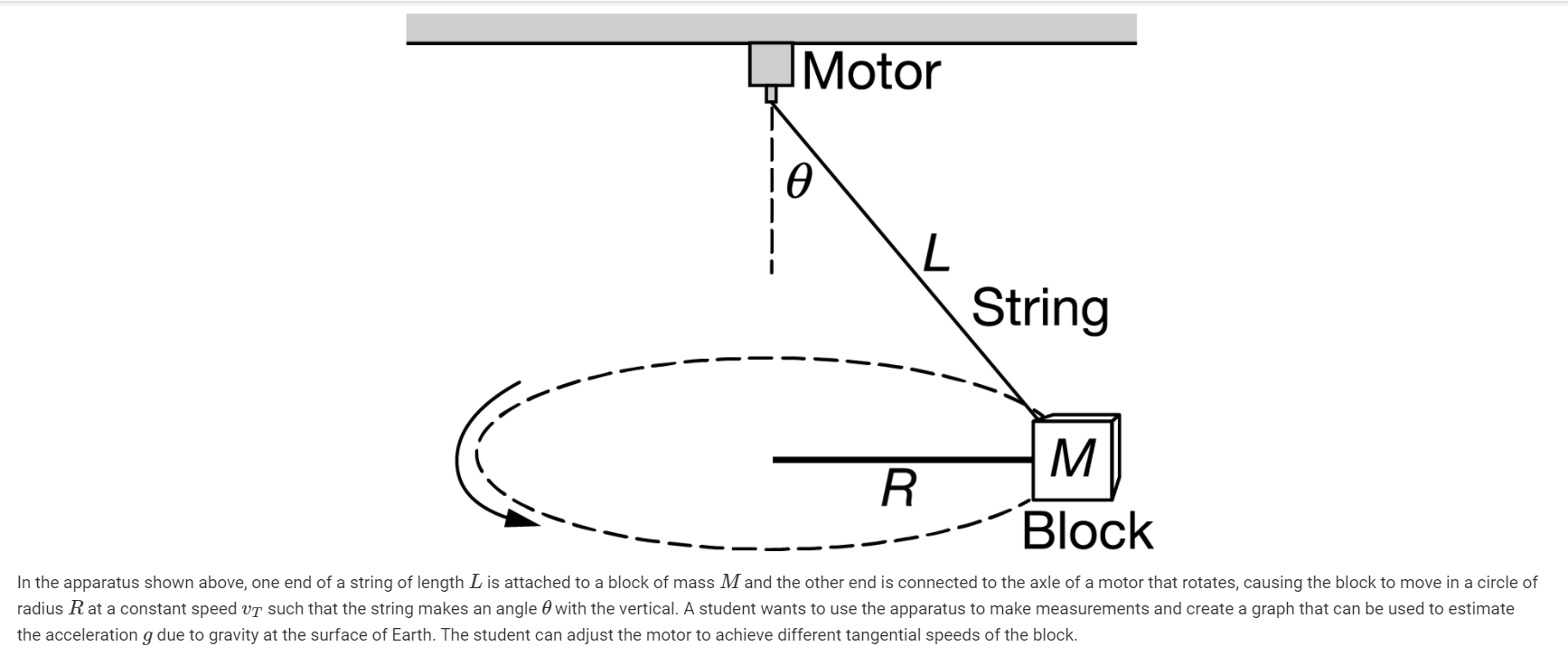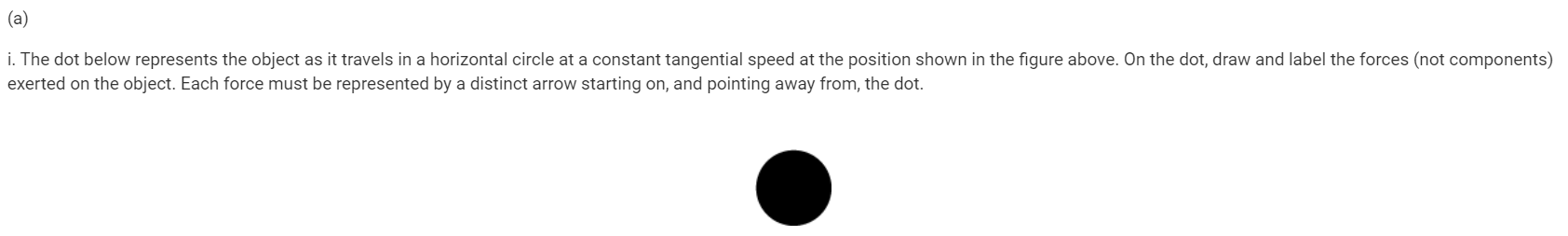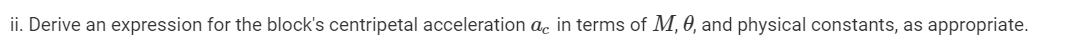# Question Solved1 AnswerMotor L String M R Block In the apparatus shown above, one end of a string of length L is attached to a block of mass M and the other end is connected to the axle of a motor that rotates, causing the block to move in a circle of radius R at a constant speed vt such that the string makes an angle with the vertical. A student wants to use the apparatus to make measurements and create a graph that can be used to estimate the acceleration g due to gravity at the surface of Earth. The student can adjust the motor to achieve different tangential speeds of the block. (a) i. The dot below represents the object as it travels in a horizontal circle at a constant tangential speed at the position shown in the figure above. On the dot, draw and label the forces (not components) exerted on the object. Each force must be represented by a distinct arrow starting on, and pointing away from, the dot. ii. Derive an expression for the block's centripetal acceleration ac in terms of M, 0, and physical constants, as appropriate.Transcribed Image Text: Motor L String M R Block In the apparatus shown above, one end of a string of length L is attached to a block of mass M and the other end is connected to the axle of a motor that rotates, causing the block to move in a circle of radius R at a constant speed vt such that the string makes an angle with the vertical. A student wants to use the apparatus to make measurements and create a graph that can be used to estimate the acceleration g due to gravity at the surface of Earth. The student can adjust the motor to achieve different tangential speeds of the block. (a) i. The dot below represents the object as it travels in a horizontal circle at a constant tangential speed at the position shown in the figure above. On the dot, draw and label the forces (not components) exerted on the object. Each force must be represented by a distinct arrow starting on, and pointing away from, the dot. ii. Derive an expression for the block's centripetal acceleration ac in terms of M, 0, and physical constants, as appropriate.
More
Transcribed Image Text: Motor L String M R Block In the apparatus shown above, one end of a string of length L is attached to a block of mass M and the other end is connected to the axle of a motor that rotates, causing the block to move in a circle of radius R at a constant speed vt such that the string makes an angle with the vertical. A student wants to use the apparatus to make measurements and create a graph that can be used to estimate the acceleration g due to gravity at the surface of Earth. The student can adjust the motor to achieve different tangential speeds of the block. (a) i. The dot below represents the object as it travels in a horizontal circle at a constant tangential speed at the position shown in the figure above. On the dot, draw and label the forces (not components) exerted on the object. Each force must be represented by a distinct arrow starting on, and pointing away from, the dot. ii. Derive an expression for the block's centripetal acceleration ac in terms of M, 0, and physical constants, as appropriate.